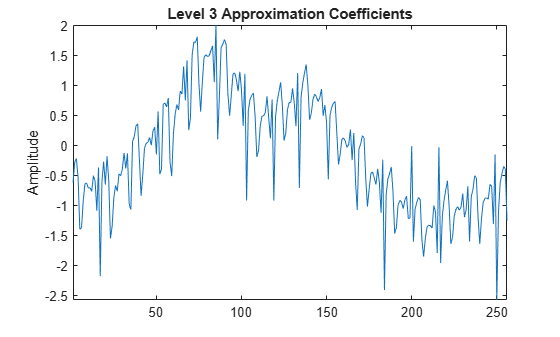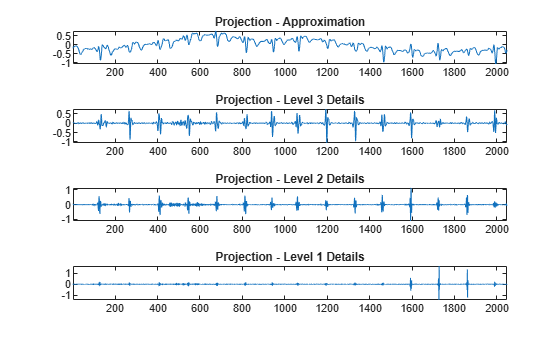# lwtcoef

Extract or reconstruct 1-D LWT wavelet coefficients and orthogonal projections

## Syntax

``y = lwtcoef(ca,cd)``
``y = lwtcoef(ca,cd,Name,Value)``

## Description

````y = lwtcoef(ca,cd)` returns the level 1 approximation coefficients that correspond to the approximation and detail coefficients, `ca` and `cd`, respectively. `ca` and `cd` are outputs of `lwt`.```

example

````y = lwtcoef(ca,cd,Name,Value)` specifies options using one or more name-value arguments. For example, ```y = lwtcoef(ca,cd,'OutputType','coefficients')``` specifies coefficients output.```

## Examples

collapse all

Load a 1-D signal of length 2048. Plot the signal.

```load wecg plot(wecg) title('Signal') ylabel('Amplitude') axis tight```Create a lifting scheme associated with the `db4` wavelet. Use the lifting scheme to obtain the wavelet decomposition of the signal to the maximum level. Confirm the length of the detail coefficients cell array equals `floor(log2(N))`, where N is the length of the signal.

```wv = 'db4'; lsc = liftingScheme('Wavelet',wv); [ca,cd] = lwt(wecg,'LiftingScheme',lsc); [length(cd) floor(log2(length(wecg)))]```
```ans = 1×2 11 11 ```

Extract and plot the approximation coefficients at level 3. Confirm the length of the extraction is one-eighth the length of the original signal.

```approxCf = lwtcoef(ca,cd,'LiftingScheme',lsc,'OutputType','coefficients','Level',3); [2048/(2^3) length(approxCf)]```
```ans = 1×2 256 256 ```
```plot(approxCf) title('Level 3 Approximation Coefficients') ylabel('Amplitude') axis tight```Obtain the orthogonal projection of the level 3 approximation coefficients. Also obtain the orthogonal projections of the detail coefficients at levels 1, 2, and 3. Plot the results.

```approx3 = lwtcoef(ca,cd,'LiftingScheme',lsc,'OutputType','projection','Level',3); det3 = lwtcoef(ca,cd,'LiftingScheme',lsc,'OutputType','projection','Level',3,'Type','detail'); det2 = lwtcoef(ca,cd,'LiftingScheme',lsc,'OutputType','projection','Level',2,'Type','detail'); det1 = lwtcoef(ca,cd,'LiftingScheme',lsc,'OutputType','projection','Level',1,'Type','detail'); subplot(4,1,1) plot(approx3) title('Projection - Approximation') axis tight subplot(4,1,2) plot(det3) title('Projection - Level 3 Details') axis tight subplot(4,1,3) plot(det2) title('Projection - Level 2 Details') axis tight subplot(4,1,4) plot(det1) title('Projection - Level 1 Details') axis tight```Confirm the sum of the four projections equals the original signal.

`max(abs(wecg-(approx3+det3+det2+det1)))`
```ans = 1.3323e-15 ```

## Input Arguments

collapse all

Approximation (lowpass) coefficients at the coarsest level, specified as a scalar, vector, or matrix. The coefficients are the output of `lwt`.

Data Types: `single` | `double`
Complex Number Support: Yes

Detail coefficients, specified as an L-by-1 cell array, where L is the level of the transform. The elements of `cd` are in order of decreasing resolution. The coefficients are the output of `lwt`.

Data Types: `single` | `double`
Complex Number Support: Yes

### Name-Value Arguments

Specify optional pairs of arguments as `Name1=Value1,...,NameN=ValueN`, where `Name` is the argument name and `Value` is the corresponding value. Name-value arguments must appear after other arguments, but the order of the pairs does not matter.

Before R2021a, use commas to separate each name and value, and enclose `Name` in quotes.

Example: ```y = lwtcoef(ca,cd,'LiftingScheme',lsc,'OutputType','coefficients','Level',2)``` uses the lifting scheme `lsc` to extract the approximation coefficients at level 2.

Orthogonal or biorthogonal wavelet, specified as a character vector or string scalar. See the Wavelet property of `liftingScheme` for the list of supported wavelets. For perfect reconstruction, the specified wavelet must match the wavelet you used to generate `ca` and `cd`.

You cannot specify `'Wavelet'` and `'LiftingScheme'` name-value arguments at the same time.

Lifting scheme to use, specified as a `liftingScheme` object. For perfect reconstruction, the specified lifting scheme must match the lifting scheme you used to generate `ca` and `cd`.

You cannot specify `'Wavelet'` and `'LiftingScheme'` name-value arguments at the same time.

Output type, specified as one of:

• `'coefficients'` — Extract the approximation or details coefficients

• `'projection'` — Return the projection (reconstruction) of the approximation or details coefficients

Example: ```y = lwtcoef(ca,cd,'OutputType','projection','Type','detail')``` returns the projection corresponding to the detail coefficients at the finest scale.

Type of coefficients to extract or reconstruct, specified as `'approximation'` or `'detail'`.

Example: `y = lwtcoef(ca,cd,'Type','detail')` extracts the detail coefficients at the finest scale.

Level of coefficients to extract or reconstruct, specified as an integer in the range `[1,N]`, where N is the length of `cd`.

Example: ```y = lwtcoef(ca,cd,'LiftingScheme',lsc,'Level',3)``` uses the lifting scheme `lsc` to extract the approximation coefficients at level 3.

Data Types: `double`

Handling integer-valued data, specified as one of these:

• `1` (`true`) — Preserve integer-valued data

• `0` (`false`) — Do not preserve integer-valued data

`Int2Int` must match the value you used to generate `ca` and `cd`.

Example: `y = lwtcoef(ca,cd,Int2Int=true)` preserves integer-valued data.

Extension mode to use to extract or reconstruct the coefficients, specified as one of these:

• `"periodic"` — Periodized extension

• `"zeropad"` — Zero extension

• `"symmetric"` — Symmetric extension

This argument specifies how to extend the signal at the boundaries. The extension mode must match the value you used to generate `ca` and `cd`.

Example: `y = lwtcoef(ca,cd,Extension="zeropad")` specifies zero extension.

## Output Arguments

collapse all

Extracted coefficients or projection, returned as a vector or matrix. If `ca` is a scalar or vector, and the elements of `cd` are vectors, then `y` is a vector. If `ca` and the elements of `cd` are matrices, then `y` is a matrix, where each column is the extraction or projection of the corresponding columns in `ca` and `cd`.

Data Types: `single` | `double`

## Version History

Introduced in R2021a

expand all

Behavior changed in R2021a